# Dealing with boundary conditions in system of ODEs

## Homework Statement

I'm trying to plot the steady state concentration of yA vs. x, yB vs x and yu vs x using centered finite difference method.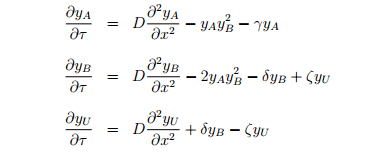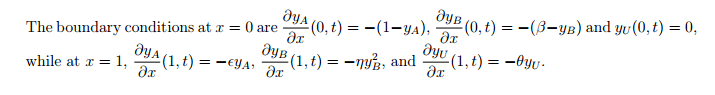## Homework Equations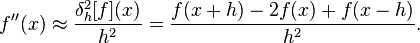## The Attempt at a Solution

τ represents the dimensionless time variable, so steady state would mean that the left hand side of each of the differential equations is 0.

I began this problem by replacing the derivatives that appear in the differential equations with the finite difference approximations for them. For example, yA: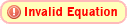I also used the boundary conditions: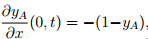but I don't know at which point, I am taking yA on the right side from. In the below equation should yA on the right side be yA,1 or something else (since the below equation estimates the derivative at the node n=1?More importantly, how do I deal with this type of boundary condition in Matlab? I am used to dealing with boundary conditions such as yA(0,t)=0 for which I can simply initialize Y(1)=0 in matlab but in this case I'm given a derivative boundary condition.

SteamKing
Staff Emeritus
Homework Helper
These aren't ODEs; they're PDEs.

Chestermiller
Mentor
What you do is add a fictitious point at -1 such that:

$$\frac{y_{1}-y_{-1}}{2h}=-(1-y_0)$$

Combining this with ##\frac{(y_{-1}+2y_0+y_1)}{h^2}## gives:

$$\frac{(y_{-1}-2y_0+y_1)}{h^2}=\frac{2(y_1-y_0)}{h^2}-\frac{2(1-y_0)}{h}$$

You solve the time-dependent equation at x = 0, and use this in that equation. This is a standard way of handling that type of flux boundary condition.

Chet

•582153236